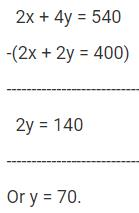Courses

# Test: Quadratic Equations - 4

## 2 Questions MCQ Test Quantitative Aptitude for GMAT | Test: Quadratic Equations - 4

Description
This mock test of Test: Quadratic Equations - 4 for GMAT helps you for every GMAT entrance exam. This contains 2 Multiple Choice Questions for GMAT Test: Quadratic Equations - 4 (mcq) to study with solutions a complete question bank. The solved questions answers in this Test: Quadratic Equations - 4 quiz give you a good mix of easy questions and tough questions. GMAT students definitely take this Test: Quadratic Equations - 4 exercise for a better result in the exam. You can find other Test: Quadratic Equations - 4 extra questions, long questions & short questions for GMAT on EduRev as well by searching above.
QUESTION: 1

### A poultry farm has only chickens and pigs. When the manager of the poultry counted the heads of the stock in the farm, the number totaled up to 200. However, when the number of legs was counted, the number totaled up to 540. How many more chickens were there in the farm? Note: In the farm, each pig had 4 legs and each chicken had 2 legs.

Solution:

Step 1 of solving this GMAT Linear Equations Question: Assign Variables and Frame Equations

Let the number of chickens in the farm be 'x'.
Let the number of pigs in the farm be 'y'.

Each pig or each chicken has one head.
Therefore, number of heads will be the same as the sum of the chickens and pigs in the farm.
The count of the heads in the farm is 200. So, the sum of the number of chickens and pigs is 200.
So, x + y = 200 .... (1)

Each chicken has 2 legs and each pig has 4 legs
'x' chickens will therefore, have 2x legs and 'y' pigs will have 4y legs.
The count of the legs in the farm is 540. So, the sum of the number of legs of chickens and the number of legs of pigs is 540.
Therefore, 2x + 4y = 540 .... (2)

Step 2 of solving this GMAT Linear Equations Question: Solve the system of linear equations

Multiply equation (1) by 2. We get 2x + 2y = 400 .... (3)
Subtract equation (3) from equation (2).Substitute 'y' as 70 in equation (1).
70 + y = 200. So, x = 130

'x' is the number of chickens in the farm. 'y' is the number of pigs in the farm. So, the farm has 130 chickens and 70 pigs.

Note:The question is "How many more chickens were there in the farm?"
The farm has 130 chickens and 70 pigs. So, the farm has (130 - 70) = 60 more chickens

Choice C is the correct answer to this linear equations word problem.

QUESTION: 2

### Three years back, a father was 24 years older than his son. At present the father is 5 times as old as the son. How old will the son be three years from now?

Solution:

Step 1 of solving this GMAT Linear Equations Question: From words to mathematical equations

Let the age of the son 3 years back be 'x' years.
Information 1: Three years back, a father was 24 years older than his son.
Therefore, the age of the father 3 years back was (x + 24).

If the age of the son 3 years back was 'x' years, the present age of the son is x + 3.
Present age of father = x + 24 + 3

Information 2: The father is at present 5 times as old as the son.
i.e., (x + 24 + 3) = 5(x + 3)
Or x + 27 = 5x + 15
Or 4x = 12 or x = 3.

Step 2 of solving this GMAT Algebra Word Problem: From unknown to the answer

x was the age of the son 3 years back.
Therefore, the son was 3 years old 3 years back.

The question is "How old will the son be three years from now?"
If the son was 3 years old, 3 years back, the son is 6 years old now.
Hence, he will be 9 years old three years from now.

Choice D is the correct answer.